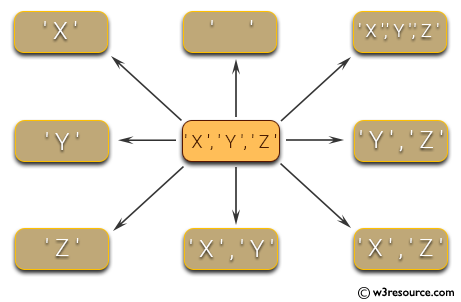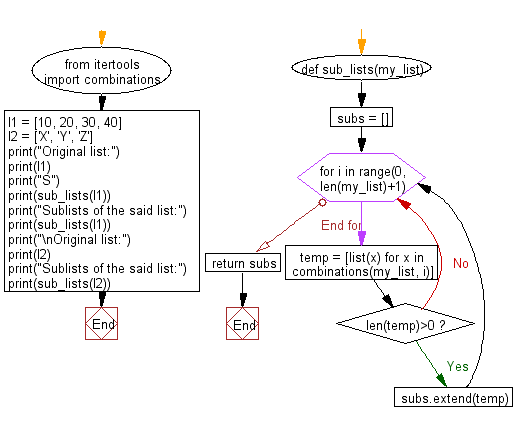﻿ Python: Generate all sublists of a list - w3resource# Python: Generate all sublists of a list

## Python List: Exercise - 33 with Solution

Write a Python program to generate all sublists of a list.Sample Solution:-

Python Code:

``````from itertools import combinations
def sub_lists(my_list):
subs = []
for i in range(0, len(my_list)+1):
temp = [list(x) for x in combinations(my_list, i)]
if len(temp)>0:
subs.extend(temp)
return subs

l1 = [10, 20, 30, 40]
l2 = ['X', 'Y', 'Z']
print("Original list:")
print(l1)
print("S")
print(sub_lists(l1))
print("Sublists of the said list:")
print(sub_lists(l1))
print("\nOriginal list:")
print(l2)
print("Sublists of the said list:")
print(sub_lists(l2))
```
```

Sample Output:

```Original list:
[10, 20, 30, 40]
S
[[], , , , , [10, 20], [10, 30], [10, 40], [20, 30], [20, 40], [30, 40], [10, 20, 30], [10, 20, 40], [10, 30, 40], [20, 30, 40], [10, 20, 30, 40]]
Sublists of the said list:
[[], , , , , [10, 20], [10, 30], [10, 40], [20, 30], [20, 40], [30, 40], [10, 20, 30], [10, 20, 40], [10, 30, 40], [20, 30, 40], [10, 20, 30, 40]]

Original list:
['X', 'Y', 'Z']
Sublists of the said list:
[[], ['X'], ['Y'], ['Z'], ['X', 'Y'], ['X', 'Z'], ['Y', 'Z'], ['X', 'Y', 'Z']]
```

Flowchart:## Visualize Python code execution:

The following tool visualize what the computer is doing step-by-step as it executes the said program:

Python Code Editor:

Have another way to solve this solution? Contribute your code (and comments) through Disqus.

What is the difficulty level of this exercise?

Test your Programming skills with w3resource's quiz.

﻿

## Python: Tips of the Day

Clamps num within the inclusive range specified by the boundary values x and y:

Example:

```def tips_clamp_num(num,x,y):
return max(min(num, max(x, y)), min(x, y))
print(tips_clamp_num(2, 4, 6))
print(tips_clamp_num(1, -1, -6))
```

Output:

```4
-1
```